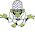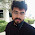### Swap two numbers using Bitwise Operator.

So why use Bitwise Operator?

In coding, if we talk about the efficiency of the program then the Bitwise operator is always more efficient than an arithmetic operator.

and the reason behind this is Bitwise operator directly works on Bits, that's why Bitwise Operator are recommended by many programmers.

so here we'll see an example of swapping two numbers using a bitwise operator, without using a third variable.

 public class JavaOneWorld {    public static void main(String args[]) {      int x=10;      int y=25;      System.out.println("Before Swap: value of x= "+x+" value of y= "+y);     x = x^y;     y = x^y;     x = x^y;      System.out.println("After Swap: value of x= "+x+" value of y= "+y);    }}

1.1.